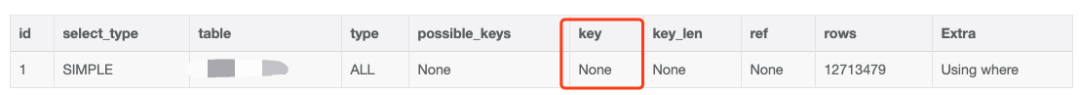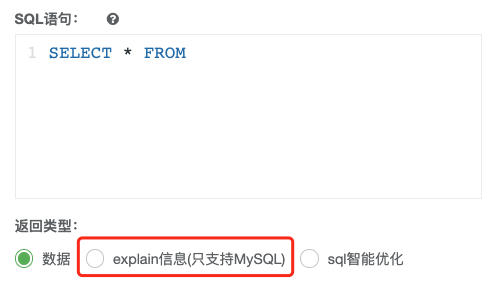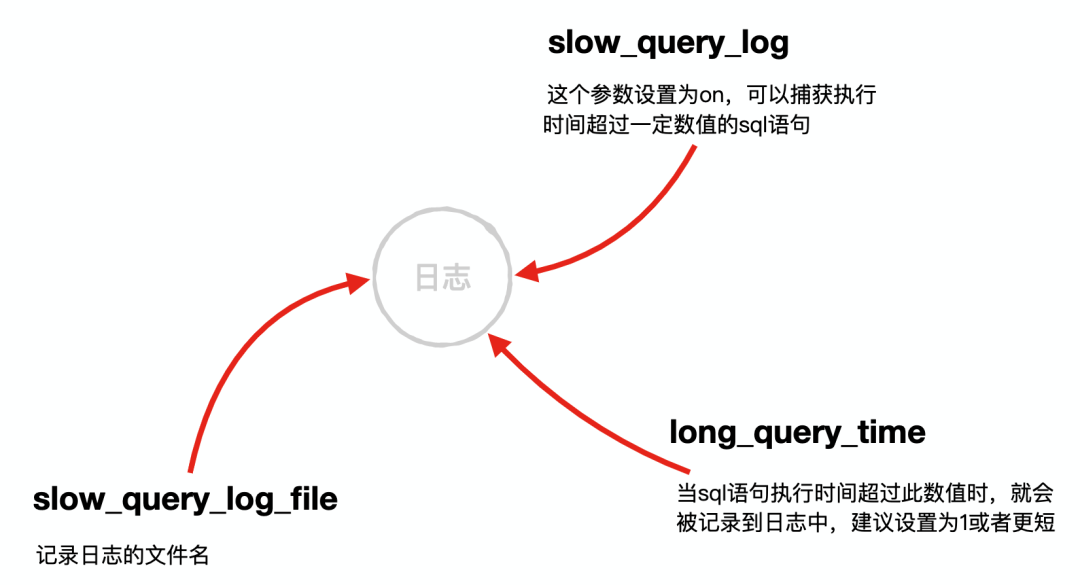# SQL 进阶技巧（下）

danielmou

0收藏

​上文​​我们简述了 SQL 的一些进阶技巧，一些朋友觉得不过瘾，我们继续来下篇，再送你 10 个技巧

``SELECT * FROM film LIMIT 100000, 10``

offset 特别大!

LIMIT 能很好地解决分页问题，但如果 offset 过大的话，会造成严重的性能问题，原因主要是因为 MySQL 每次会把一整行都扫描出来，扫描 offset 遍，找到 offset 之后会抛弃 offset 之前的数据，再从 offset 开始读取 10 条数据，显然，这样的读取方式问题。

``SELECT <cols> FROM profiles where sex='M' order by rating limit 100000, 10;``

``````SELECT <cols>
FROM profiles
inner join
(SELECT id form FROM profiles where x.sex='M' order by rating limit 100000, 10)
as x using(id);``````

``````○ SELECT * FROM SomeTable WHERE col_1 = 10 AND col_2 = 100 AND col_3 = 500;
○ SELECT * FROM SomeTable WHERE col_1 = 10 AND col_2 = 100 ;
× SELECT * FROM SomeTable WHERE col_2 = 100 AND col_3 = 500 ;``````

``````× SELECT * FROM SomeTable WHERE col_1 LIKE '%a';
× SELECT * FROM SomeTable WHERE col_1 LIKE '%a%';
○ SELECT * FROM SomeTable WHERE col_1 LIKE 'a%';``````

``````SELECT
first_name,
last_name,
homeroom_nbr
FROM Students
WHERE homeroom_nbr LIKE 'A-1%';``````

``````SELECT first_name, last_name
homeroom_nbr
FROM Students
WHERE homeroom_nbr LIKE 'A-1__'; --模式字符串中包含了两个下划线``````

``SELECT COUNT(*) FROM SomeTable``

SELECT * 会提取出一整行的数据，如果查询条件中用的是组合索引进行查找，还会导致回表（先根据组合索引找到叶子节点，再根据叶子节点上的主键回表查询一整行），降低性能，而如果我们所要的数据就在组合索引里，只需读取组合索引列，这样网络带宽将大大减少,假设有组合索引列 (col_1, col_2)

``````SELECT col_1, col_2
FROM SomeTable
WHERE col_1 = xxx AND col_2 = xxx``````

``````SELECT *
FROM SomeTable
WHERE col_1 = xxx AND  col_2 = xxx``````

``````SELECT *
FROM  SomeTable
WHERE `status` = 0
AND `gmt_create` > 1490025600
AND `gmt_create` < 1490630400
AND `id` > 0
AND `post_id` IN ('67778', '67811', '67833', '67834', '67839', '67852', '67861', '67868', '67870', '67878', '67909', '67948', '67951', '67963', '67977', '67983', '67985', '67991', '68032', '68038'/*... omitted 480 items ...*/)
order by id asc limit 200;``````

post_id 也加了索引，理论上走 post_id 索引会很快查询出来，但实现了通过 EXPLAIN 发现走的却是 id 的索引（这里隐含了一个常见考点，在多个索引的情况下, MySQL 会如何选择索引），而 id > 0 这个查询条件没啥用，直接导致了全表扫描， 所以在有多个索引的情况下一定要慎用，可以使用 force index 来强制走某个索引，以这个例子为例，可以强制走 post_id 索引，效果立杆见影。``````-- 批量插入
INSERT INTO TABLE (id, user_id, title) VALUES (1, 2, 'a'),(2,3,'b');``````

``````INSERT INTO TABLE (id, user_id, title) VALUES (1, 2, 'a');
INSERT INTO TABLE (id, user_id, title) VALUES (2,3,'b');``````### 总结帖子
视频
声望
粉丝
相关问题
社区精华内容